# Top 21 VLOOKUP Tips and Tricks for Consultants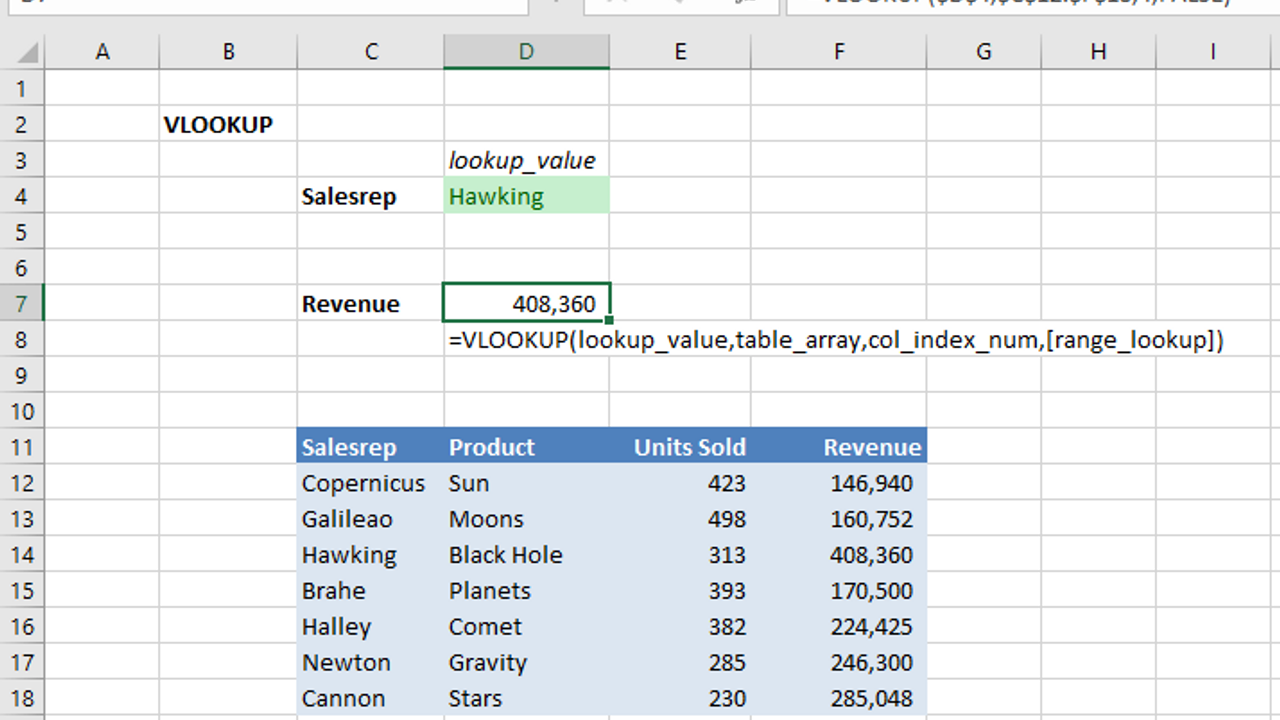You’re on a consulting project where you need to compare, analyze, and recalculate thousands of items in a list spit out from the client’s massive database. It could take you until June two years from now to do it manually, or you could learn a few tips and tricks on how to use VLOOKUP and get your consulting project done in an afternoon.

Here is how to use VLOOKUP to find, compare, and analyze list data quickly.

Use VLOOKUP to find and retrieve data from an Excel list. This list of the top 21 best VLOOKUP tips will take you from novice to master in retrieving data from Excel lists. You can use VLOOKUP to,

• Look up prices, names, or regions from a list
• Use one item to find related items in a list
• Create one list from two related lists
• Find near matches in a list
• Scan the titles of these tips to see how you can increase your Excel performance. This figure shows one of the tips you will find in the list, Using VLOOKUP with Multiple Criteria from Two Drop-Down Lists.Look up data using multiple criteria by combining VLOOKUP with drop-down lists.

## 1. VLOOKUP Basics and Free Tutorials

This is the most basic VLOOKUP tip, before working on advanced VLOOKUP tips you must know the basics of how VLOOKUP works! Use VLOOKUP to find and retrieve data from an Excel list. VLOOKUP looks down the leftmost (Vertical) column in an Excel list until it finds the row containing the value you are looking for. (Warning: It stops looking when it finds the first instance.) Then, VLOOKUP looks across that row to the column you specify to find the data in that column you want to retrieve.

VLOOKUP’s syntax is,

VLOOKUP(lookup_value,table_array,col_index_number,[range_lookup])

The lookup_value is the value you want to find in the leftmost column of the Excel list. table_array is the Excel list or Excel Table. col_index_number is the number of the column in the list containing the data to be want retrieved. The first column is 1. Set the optional parameter [range_lookup] to TRUE if you want an approximate match to the lookup_value or FALSE if you want an exact match.

Beware, if you do not enter [range_lookup] it defaults to TRUE, an approximate match. This can cause invalid returns.

The leftmost column in the list/table where VLOOKUP looks for a match to [lookup_array] must be sorted in ascending order. If it is not in ascending order VLOOKUP may return invalid data.

For a great explanation of how VLOOKUP works, go to the Excel Campus by Jon Acampora and see his example using a Starbucks menu.

Microsoft has a couple of great free video tutorials on When and how to use VLOOKUP.

## 2. Use VLOOKUP to Retrieve Data from a List or Table

The easiest and most frequent use of VLOOKUP is to retrieve data from a list. In this example, the name Hawking is the value being searched for in the leftmost column. The Revenue data is retrieved from the fourth (4) column.Use VLOOKUP to easily retrieve data from a list.

## 3. Use Absolute References for lookup_value and table_array

It is always a good practice to use an absolute reference as the table_array in the VLOOKUP function. Whenever the lookup_value is in a single cell, then the reference to that cell should also be an absolute reference.

Do not use an absolute reference for the lookup_value if you are copying VLOOKUP down a list. This happens when you want to use VLOOKUP to lookup data from one table and bring the found data into a column in another table. In this case, you will want to make sure lookup_value is an absolute reference or named range.

## 4. Make VLOOKUP Easy to Read and Maintain by Using Range Names

This VLOOKUP tip makes it easier to read and maintain your formulas. Use named ranges in the VLOOKUP function so parameters inside a VLOOKUP function are easier to understand. For example, the function,

=VLOOKUP(rSalesRep,tblRegionalSales,5,FALSE)

is easy to read as the function is looking for the Sales Rep’s name in the range rSalesRep of the table tblRegionalSales. It will find the exact match (specified by FALSE) in column 5 of the table tblRegionalSales.

The function with named ranges is much easier to read than,

=VLOOKUP(\$B\$25,\$F\$53:\$O\$312,5,FALSE)

Since named ranges are normally created as absolute references, using named ranges also helps maintain VLOOKUP.

## 5. Use F5 to Insert a Named Range or Excel Table Name in the VLOOKUP Function

This VLOOKUP tip is a great time saver and guardian against errors. To enter the lookup_value or table_array in the VLOOKUP function, move the insertion point in the function to where you would type a named range, then press F5. The Go To dialog box displays showing a list of range names and Excel Table names. Select the name you need and press Enter to insert the name in the function.

In this figure, the range name, tblUnitSalesbyRegion, has already been entered from the Go To dialog box.Use the F5 key, Go To, to go to a range name or to insert the range name in a function.

When entering a range in a function, press F5 to display the Go To dialog box, then select and enter the range or Table name from within the dialog box.

## 6. Use VLOOKUP with Data Validation to Make a Drop-Down List for Extracting Data

Combine VLOOKUP with a Data Validation list to give users a drop-down list of valid items they can search for. This makes it easier for users and reduces errors.

This example shows how to use Data Validation as a drop-down of available choices from the leftmost column in the list. As soon as the user selects from the Data Validation list VLOOKUP retrieves the matching data.In this figure, the Data Validation list selected and shown in C16 causes all the VLOOKUP functions in D16:I16 to show the appropriate value from the range named tblUnitSalesbyRegion, C20:I24. Selecting from the Data Validation drop-down in C16 gives VLOOKUP the value it needs to fill in the Jan through Jun data that creates the chart.

## 7. Use VLOOKUP to Join Two Tables

Use VLOOKUP to join two tables if they each have a column with the same data and the data in one of the lookup columns is unique.

For example, a table containing a product price list normally has a unique product identifier for each product. A corresponding table with customer orders would have multiple instances of the product identifier due to multiple orders of the same product.

### Merging or Joining Excel Lists

To see an example of how to merge data from two Excel lists together watch this short video that steps you through the basics, How Can I Merge or Join Two Lists on Separate Sheets?

### Merging or Joining Excel Tables

If you defined your lists as Excel Tables then joining them with VLOOKUP is almost the same, however, the table references use a different syntax from range names. In this example, Excel uses references for the Excel Table and the columns. Microsoft has a tutorial titled How Can I Merge Two or More Tables?

## 8. Use VLOOKUP with an Approximate Match for Tax Rates, Commission Rates, Shipping Rates, etc.

Most rate tables, such as tax rates, commission rates, and shipping rates will not have a value in the leftmost column that exactly matches what the user is searching for. For example, a shipping rate table may jump from 8 ounces to 16 ounces implying that anything shipped weighing between 8 and 16 ounces should ship at the 8-ounce rate.

This is where you want to use TRUE for [range_lookup]. TRUE restricts VLOOKUP so that if you have a 10-ounce package VLOOKUP returns the rate for 8-ounces. It finds the closest match without exceeding the next value in the leftmost column.

Even though TRUE specifies an approximate match, the leftmost column must still be in sorted order.

## 9. Use VLOOKUP with Wildcards for Better Approximate Matches to Words or Alphanumeric Values

Using TRUE or blank as the last parameter, [range_lookup], enables VLOOKUP to look up an approximate match. While this is helpful with lists containing numeric ranges, like the shipping rates, it does not work well when matching against words or alphanumeric lists.

Instead, use wildcards for approximate matches with text. Wildcards in VLOOKUP only work with exact matches, so be sure to use FALSE as the [range_lookup].

The wildcards asterisk, *, and question mark, ?, can be used in Excel with find/replace, SEARCH, MATCH, VLOOKUP, and HLOOKUP to find matches to a pattern that uses the wildcard.

In the lookup_value use an asterisk (*) to match one or more characters. Use a question mark (?) to match any character in that same position in lookup_value. For example, the lookup_value of,

“Jo*” returns the first match that starts with Jo, such as Johnson

“*son” returns the first match that ends with son, such as Thompson

“Sm?th” returns the first match where any letter is the third letter and the other letters match, such as Smyth

“?????” returns the first match having only five characters, such as Smith

## 10. Use VLOOKUP with Multiple Criteria by Creating a New “Helper” Column

Some of the data you work with will require two or more columns to uniquely identify what you are searching for. For example, you may have two sales people with the same last name. You need to include the first name to tell them apart. (In this case, since the lookup_values will be unique and VLOOKUP is looking for an exact match, the leftmost column does not need to be in ascending order.)

In this VLOOKUP tip, the data from two or more columns create unique lookup_values. In a new column to the left of the table, create a “helper” column containing the concatenated first and last names. Use the & to concatenate the first and last names into unique values.

Use a Helper column to concatenate two or more fields when you need to use VLOOKUP with mulitple criteria.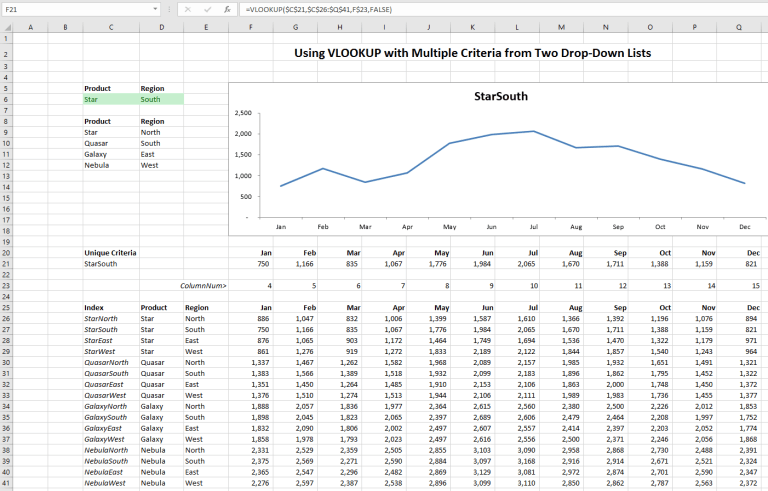Click here to see the Using VLOOKUP with Multiple Criteria from Drop-Down Lists tutorial.

Another example of using the helper column is from ExcelJet. The helper column uses & to concatenate First and Last names into a new unique value. The lookup_value in VLOOKUP is a concatenation (joining) of C3&D3. The new “helper” column is in column B.

For a step-by-step explanation check out VLOOKUP with Two or More Criteria in ExcelJet.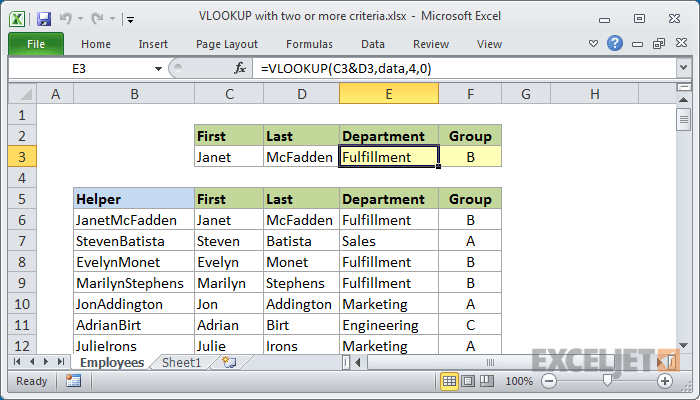ExcelJet shows how to use VLOOKUP to find data with multiple criteria by concatenating criteria.

## 11. Use VLOOKUP to Retrieve from the Nth Match

This powerful technique works around VLOOKUP’s limitation of only returning the first value and finds the Nth match in a VLOOKUP.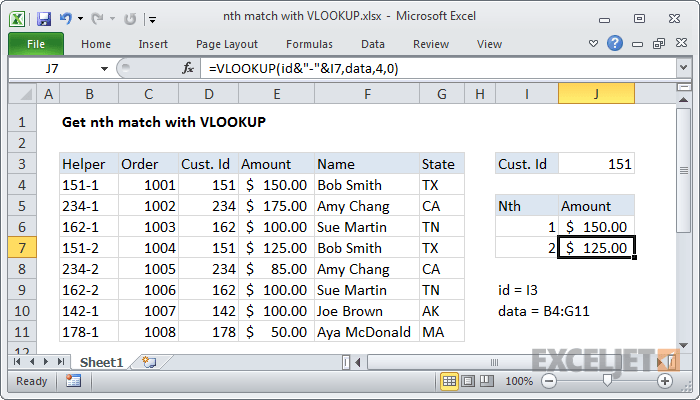Bust out of VLOOKUP’s limit of finding the first match and find the Nth match.

Finding the Nth match in a VLOOKUP is not that easy unless you know this trick. ExcelJet shows two methods to solve this limit to VLOOKUP. The first method uses a “helper” column shown in column B. The second method, not shown, uses an array formula with INDEX and MATCH. The figure below from ExcelJet shows the “Helper” column displaying the Cust. Id with a Nth number concatenated (joined) for multiple instances of Cust. Id. Learn more about this cool trick at ExcelJet’s Get Nth match with VLOOKUP.

## 12. Create a Powerful Two-Way VLOOKUP that Finds Data by Row and Column

A two-way lookup gives users the ability to find data given a value from the leftmost row and a column title. The row is found by using a lookup_value in VLOOKUP to match down the leftmost column of the list. The column from which data is retrieved is identified by using MATCH to find the column header that matches the desired title.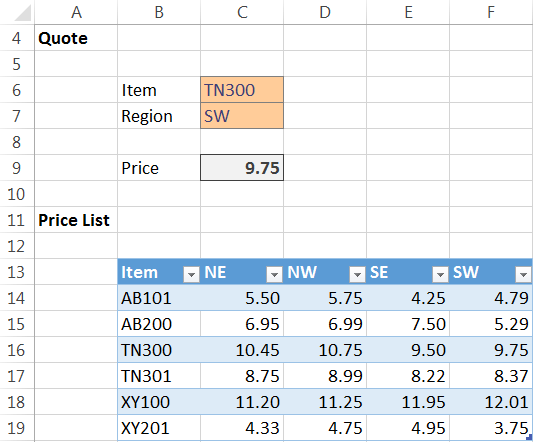Excel University shows how to use VLOOKUP and MATCH to do two-way look ups.

Excel University shows an example where the Item number finds the row and the Region finds the column. Excel’s MATCH function looks across the column headers, B13:F13, to find the col_index_num value used as the col_index_num. For full details see, Two-Dimensional VLOOKUP at Excel University.

## 13. Understand When to Use VLOOKUP, HLOOKUP, INDEX, MATCH, or OFFSET

Don’t limit yourself to thinking about retrieving data using only VLOOKUP. Excel gives you many ways to retrieve data from lists and tables. This table can help you select which function you want to use to retrieve data. There’s a lot of variety, power, and flexibility in these different approaches.

INDEX and MATCH are more powerful and flexible than VLOOKUP. To learn more about when to use them search for the blogs in Critical to Success on INDEX and MATCH.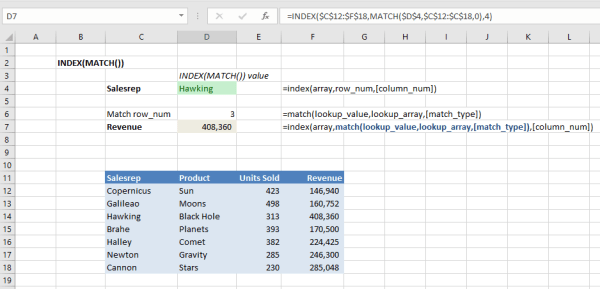It is important to know when to use VLOOKUP and when to use the INDEX and MATCH combination.

## 14. Use INDEX and MATCH as a Far More Powerful Version of VLOOKUP

VLOOKUP does its lookup down the leftmost column. Use INDEX and MATCH when you need a far more powerful version of VLOOKUP that works around this issue. MATCH finds the item you are looking for in any row or column, not just the leftmost column as in VLOOKUP. Once you’ve found the row or column number containing the match, use INDEX to retrieve the data.

Combining INDEX and MATCH gives you a very powerful set of functions for finding and retrieving data anywhere in a list. If you are unfamiliar with INDEX and MATCH, start with this Excel tutorial in Critical to Success, Using INDEX and MATCH to Retrieve Excel Data.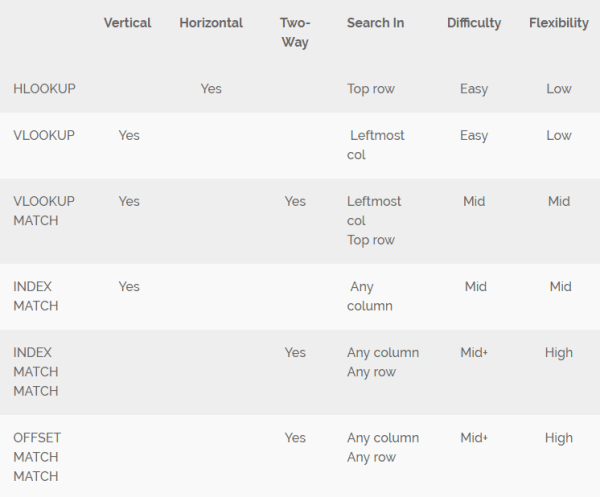INDEX and MATCH are a powerful combination that has more flexibility than VLOOKUP.

## 15. Use INDEX to Scroll Excel Charts through Time. Use this Same Trick to Scroll VLOOKUP.

While this is not a VLOOKUP function, there are many times when you will want to use INDEX and MATCH to replicate and surpass what you can do with VLOOKUP. The blogs in Critical to Success on Gantt charts and scrolling timelines are good examples of how INDEX and MATCH can find data, scroll through the data, and produce a scrolling chart.

Using a scrolling bar to change the value of the column where data is retrieved causes the data and chart to animate across time. This same technique could be used with VLOOKUP to change the column_index_num so it scrolls across time.

## 16. VLOOKUP Fails to Lookup Data to the Left. Use INDEX and MATCH instead.

VLOOKUP cannot retrieve data from columns to the left of the leftmost column in table_array. If you want to use a lookup_value on any column in table_array and retrieve data from any column in the list or table, then use the INDEX and MATCH combination described in the previous tip. For more information, see tips 13 and 14.

## 17. Understand Errors Returned by VLOOKUP

VLOOKUP returns different error codes depending upon whether you used TRUE (approximate) or FALSE (exact) as the [range_lookup] parameter. The errors returned can be,

#N/A when the the lookup_value is not found in the leftmost column of table_array.

#REF when the value for column_index_num is greater than the number of columns in table_array. This might happen if you delete columns from the table and the column_index_num is no longer valid.

#VALUE when the the value of column_index_num is less than 1. While this sounds impossible, it could occur if you are calculating the column_index_num.

Invalid Data This is the most insidious error because these errors return values, but the values may be wrong. If you are using the approximation method, then it is even harder to find errors because the error values may be close to the correct values. The best method of preventing this is by validating the formula visually checking lookup_values that include the lowest, highest, and multiple mid-points.

## 18. Give a Friendly Error Warning When VLOOKUP Fails

If VLOOKUP uses FALSE to specify an exact match the function returns #N/A in the cell when it can’t find an exact match. This can be very frustrating to inexperienced Excel users. Other errors returned can be #REF and #VALUE.

A good way to make these errors more user-friendly is to wrap VLOOKUP inside an IFERROR function so that when VLOOKUP returns an error the IFERROR displays a user-friendly text message. For example,

=IFERROR(VLOOKUP(\$D\$4,\$C\$12:\$F\$18,4,FALSE),”This is not a valid entry.”)

## 19. Avoid these Common Errors in VLOOKUP

There are a number of ways VLOOKUP can be misused and return errors or incorrect data.

VLOOKUP is not case sensitive.

VLOOKUP can return the wrong data if the leftmost column is not sorted in ascending order and [range_lookup] is TRUE or not entered. VLOOKUP will work correctly if all of the leftmost values are unique and [range_lookup] is FALSE (exact).

VLOOKUP returns an approximate match if you don’t specify TRUE as the [range_lookup].

VLOOKUP can return a mismatch if the leftmost column contains duplicate values of what you are searching for. Each value in the leftmost column must be unique unless you are using the Nth lookup tip described earlier. If there are multiple items that are the same, then VLOOKUP only returns data corresponding to the first match.

VLOOKUP may return an error if you insert a column through the table_array. Inserting a column through the table shifts the columns in the table so the col_index_num is no longer the column from which you want to retrieve data.

## 20. Alert Users to VLOOKUP Errors with Conditional Formatting

In lists containing a large number of VLOOKUP values, for example, a long customer purchase list, users may not see the error #N/A or the friendlier “This is not a valid entry.” described in tip 17. You can make errors stand out by selecting the column containing the VLOOKUP and applying a conditional format that changes the content to RED if it is #N/A or “This is not a valid entry”.

## 21. Use this VLOOKUP Troubleshooting Quick Reference from Microsoft

Download the VLOOKUP Troubleshooting Quick Reference Card from Microsoft for quick tips on troubleshooting VLOOKUP.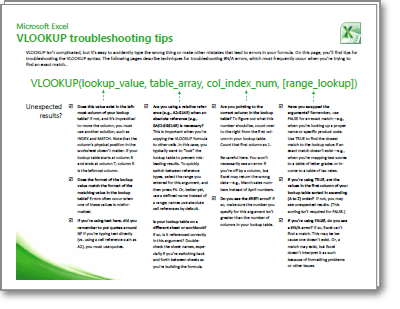#### Strategies, Tactics, and Tips for Consultants and Independent Professionals

Stay up to date with the latest opportunities, strategies, tactics, and tips for
independent consultants and professionals.

We hate SPAM. We will never sell your information, for any reason.